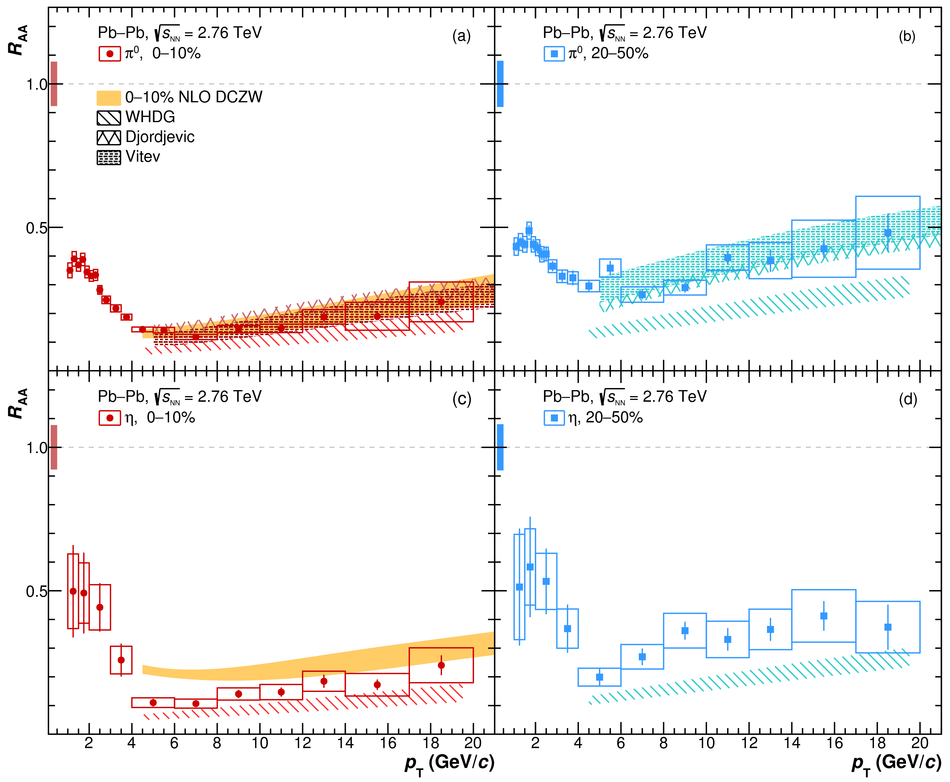Neutral pion and $η$ meson production at mid-rapidity in Pb-Pb collisions at $\sqrt{s_{NN}}$ = 2.76 TeV

Neutral pion and $\eta$ meson production in the transverse momentum range 1 <~ $p_{T}$ <~ 20 GeV/$c$ have been measured at mid-rapidity by the ALICE experiment at the Large Hadron Collider (LHC) in central and semi-central Pb-Pb collisions at $\sqrt{s_{NN}}$ = 2.76 TeV. These results were obtained using the photon conversion method as well as the PHOS and EMCal detectors. The results extend the upper $p_{T}$ reach of the previous ALICE $\pi^{0}$ measurements from 12 GeV/$c$ to 20 GeV/$c$ and present the first measurement of $\eta$ meson production in heavy-ion collisions at the LHC. The $\eta/\pi^{0}$ ratio is similar for the two centralities and reaches at high $p_{T}$ a plateau value of 0.457 $\pm$ 0.013$^{stat}$ $\pm$ 0.018$^{syst}$. A suppression of similar magnitude for $\pi^{0}$ and $\eta$ meson production is observed in Pb-Pb collisions with respect to their production in pp collisions scaled by the number of binary nucleon-nucleon collisions. We discuss the results in terms of NLO pQCD predictions and hydrodynamic models. The measurements show a stronger suppression with respect to what was observed at lower center-of-mass energies in the $p_{T}$ range 6 <~ $p_{T}$ <~ 10 GeV/$c$. At $p_{T}$ <~ 3 GeV/$c$, hadronization models describe the $\pi^{0}$ results while for the $\eta$ some tension is observed.

Figures

Figure 1

 Invariant mass distribution of reconstructed photon pairs $M_{\gamma\gamma}$ for the \pai and \e mesons measured with PCM, (a) and (b), and EMCal, (c) and (d), in the centrality class 0--10\%. The black histograms show the signal before background subtraction while the red bullets show the signal after subtraction. The estimated background is indicated by the grey dashed lines. The blue lines are the fit to the invariant mass peak after the combinatorial and residual background subtraction (see text for description).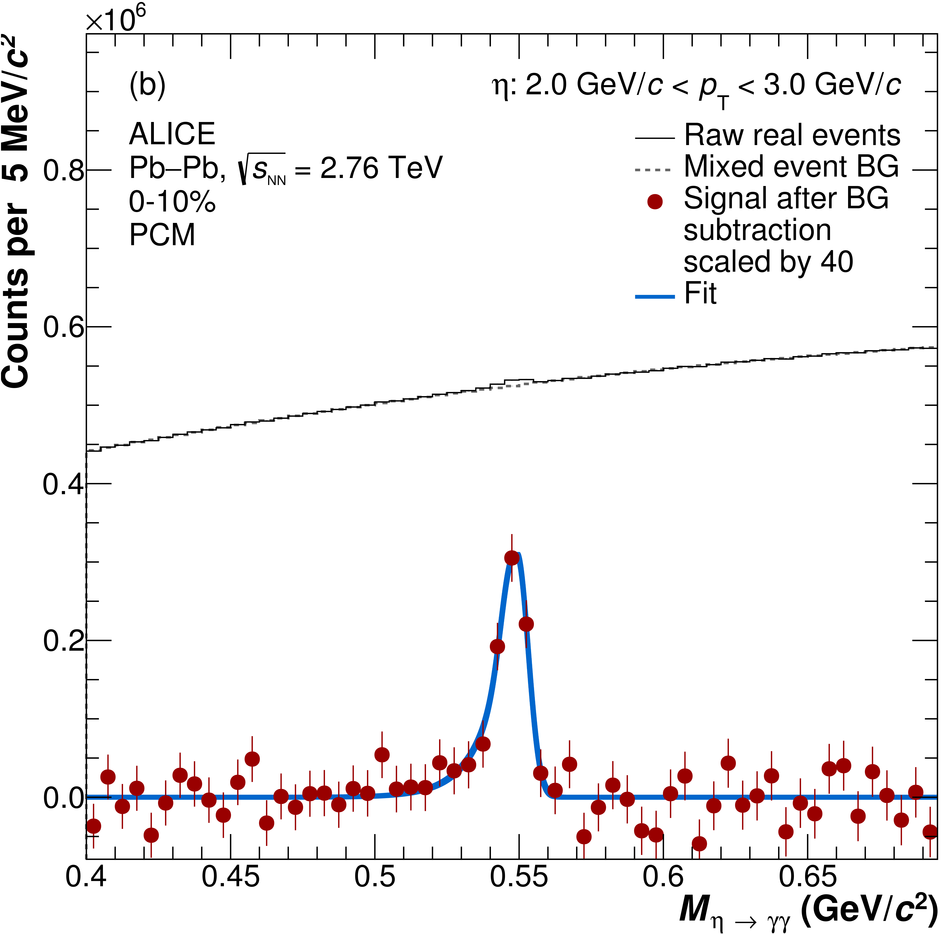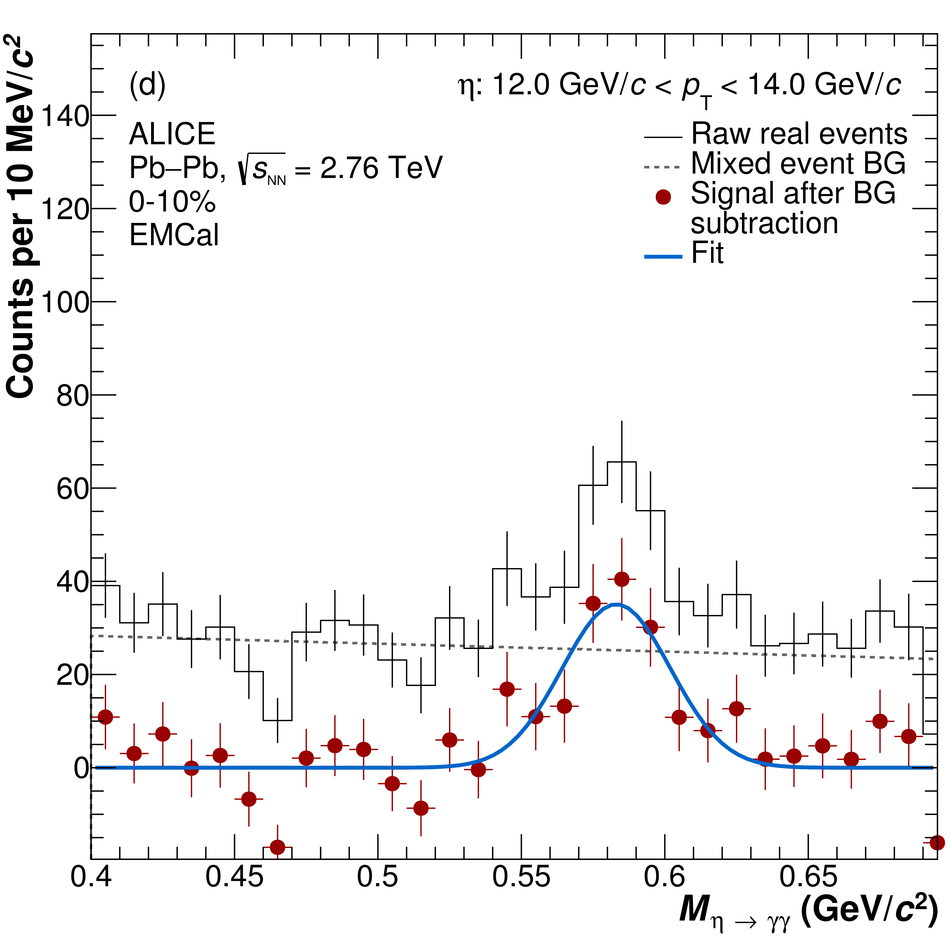Figure 2

 Invariant yields of the (a) \pai and (b) \e meson in the centrality classes 0--10\% (circles) and 20--50\% (squares). The vertical error bars represent the statistical uncertainties while the boxes represent the systematic uncertainties. The \Pb measurements are compared with the corresponding pp invariant cross sections (stars) measured at the same center-of-mass energy~. The dashed black lines correspond to the fits to the data with the two-component function. See Table~\ref{FitParam} and corresponding text for details.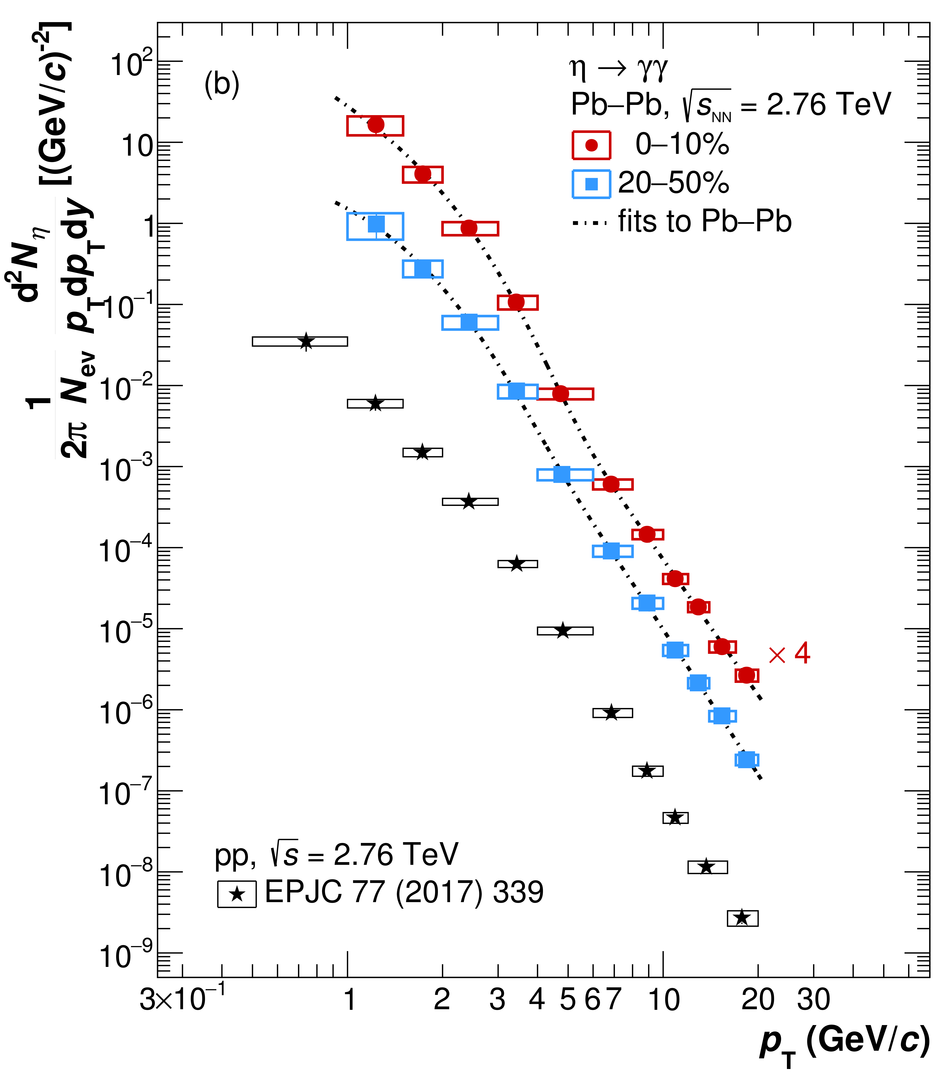Figure 3

 (a) \etapai ratio in the two centrality classes measured, 0--10\% (circles) and 20--50\% (squares). (b) Comparison of the \etapai measurement in the 0--10\% centrality class (full circles) to the corresponding ratio in pp collisions~ (stars) and to the $K^{\pm}/\pi^{\pm}$ measurement in the same centrality class, system and collision energy~ (open circles).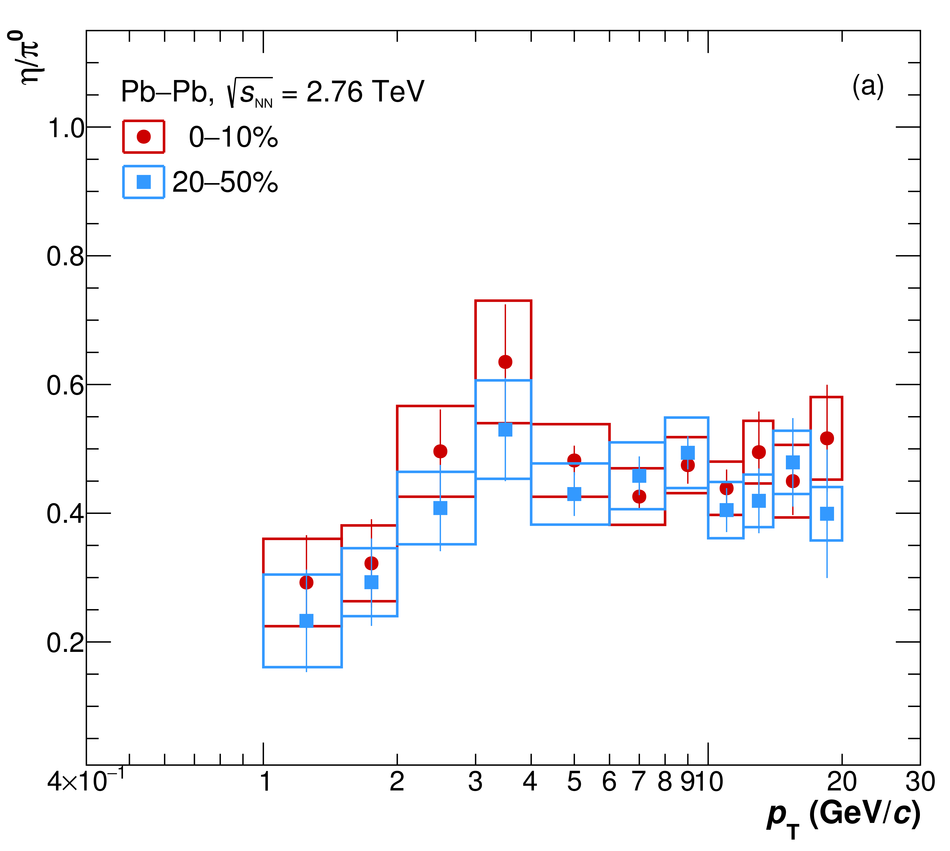Figure 4

 Measured nuclear modification factor for the \pai (empty symbols) and \e meson (full symbols) in the (a) 0--10\% and (b) 20--50\% centrality classes, compared to ALICE $\pi^{\pm}$ and $K^{\pm}$~ (open and full diamonds) in the same centrality classes. The boxes around unity represent quadratic sum of the uncertainty on $\langle \TAA \rangle$ and on the pp spectrum normalization uncertainty.Figure 5

 \RAA of the (a) \pai and (b) \e meson compared to data from lower center-of-mass energy results~.Figure 6

 (a) Comparison of the \pai (open symbols) and \e (closed symbols) meson invariant \pT-differential yields to EQ and NEQ SHM~ and to EPOS~ for the two centrality classes measured. (b$-$e) Ratio of data and theory calculations to the fit of the \pai and \e invariant yields (see Fig.~\ref{fig:InvYieldsDataOnly} and the left plot of this figure) in the two centrality classes measured. Black points show the data to fit ratio (vertical lines for statistical errors and boxes for systematic errors). The bold lines correspond to the ratio of the EQ and NEQ SHM predictions~ to the data fit, while the thin dashed lines correspond to the same ratio for the EPOS predictions~.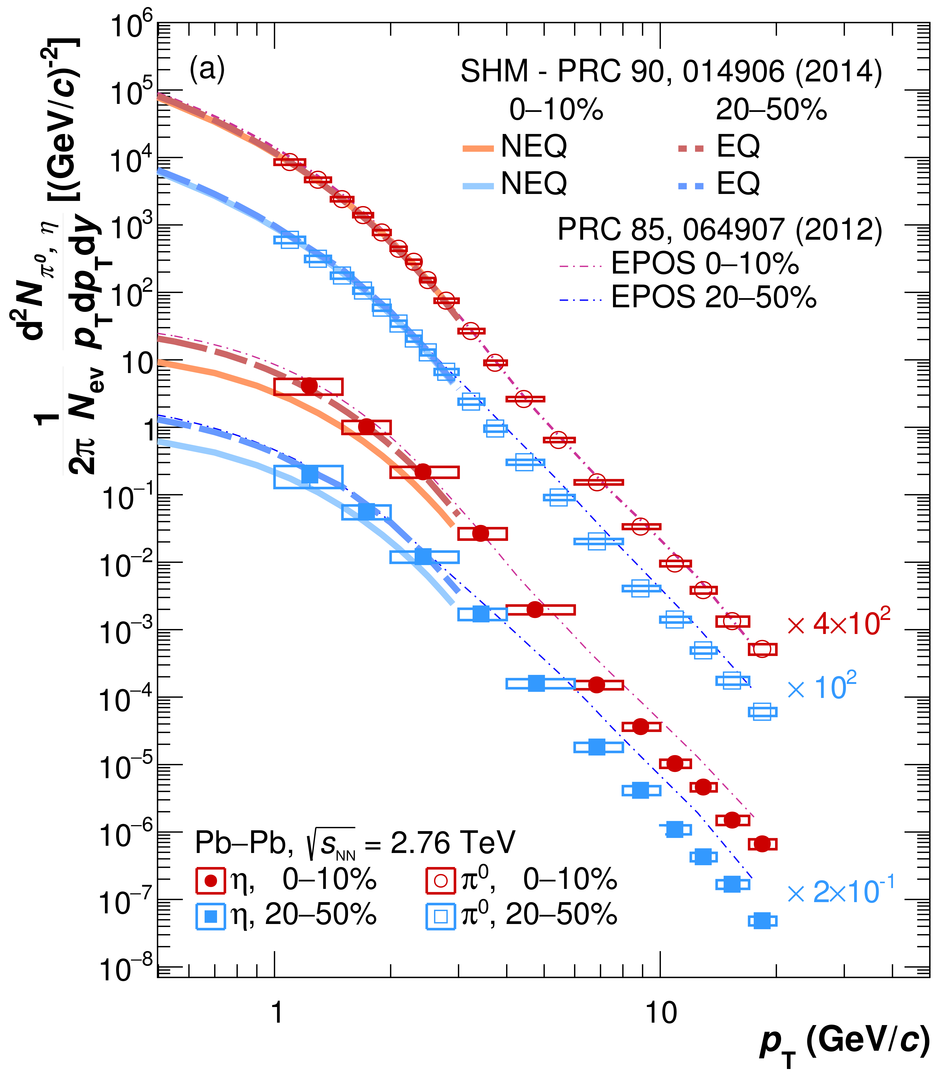Figure 7

 Comparison of the \etapai ratio in the centrality class 0--10\% (circles) to the NLO pQCD prediction by DCZW (solid band)~ and to the EQ and NEQ SHM~ and EPOS~ predictions for the input yields (bold and thin dashed lines respectively).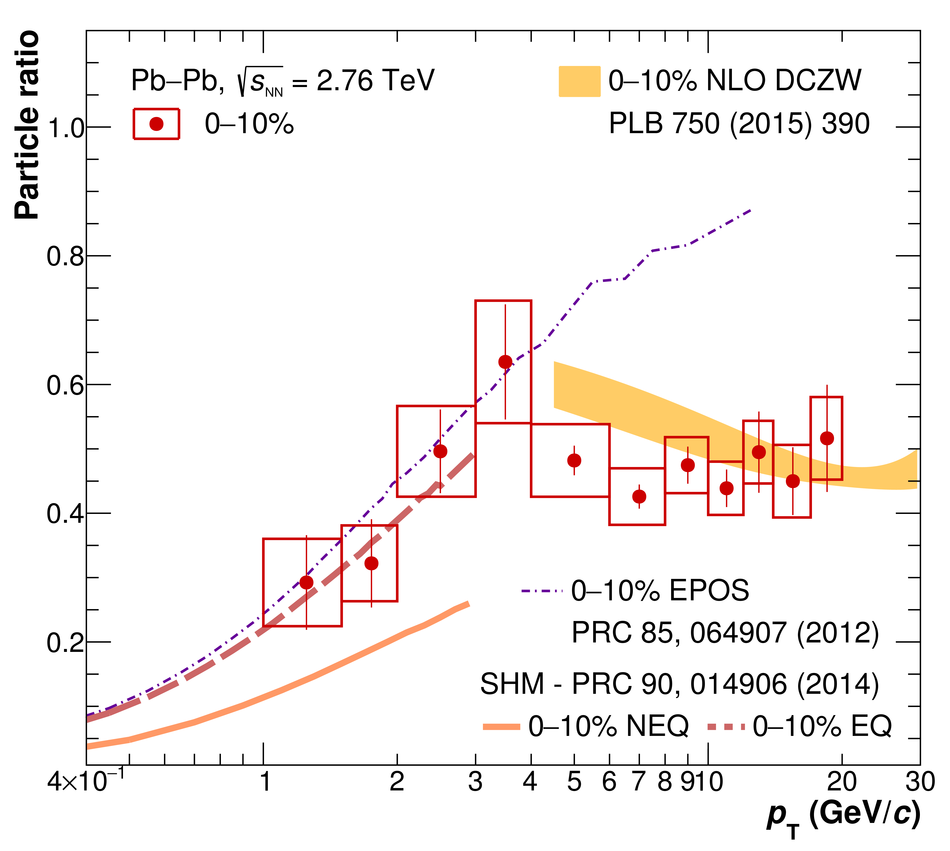Figure 8

 \RAA of (a, b) \pai and (c, d) \e meson compared to NLO pQCD predictions by DCZW (solid bands)~, by WHDG (dashed bands)~, Djordevic~\emph{et al.}~ (crossed bands, \pai only) and Vitev~\emph{et al.}~ (empty bands, \pai only) in the two centrality classes measured.